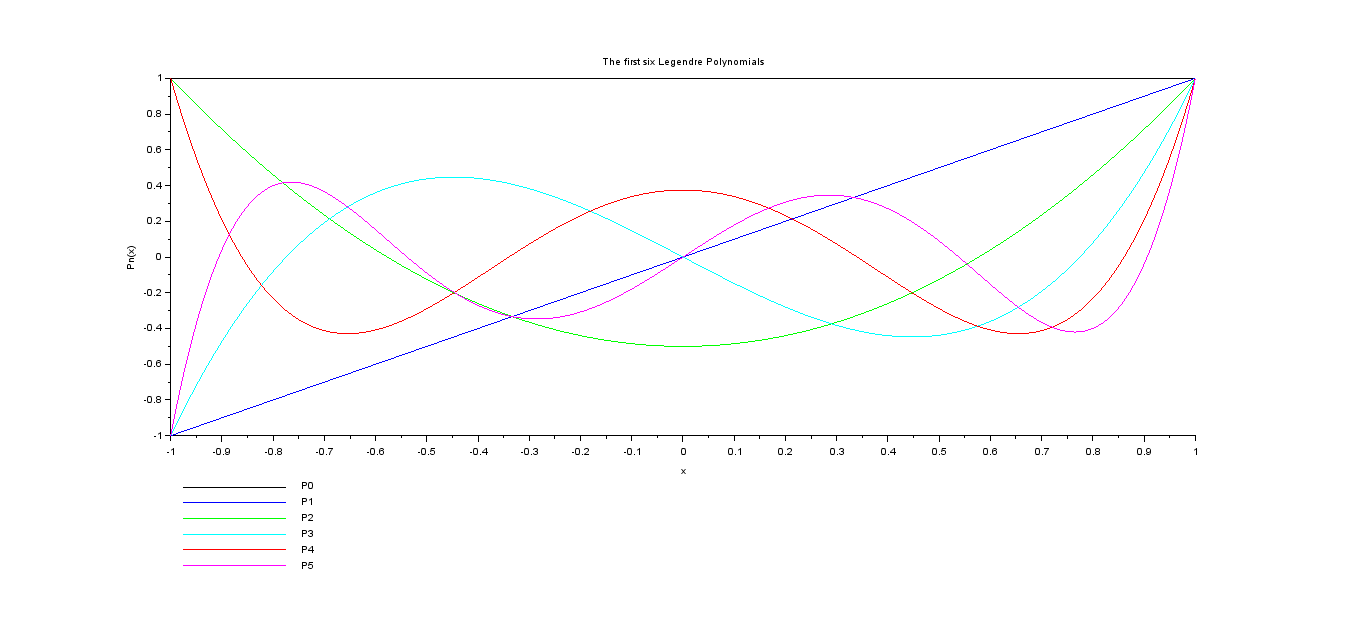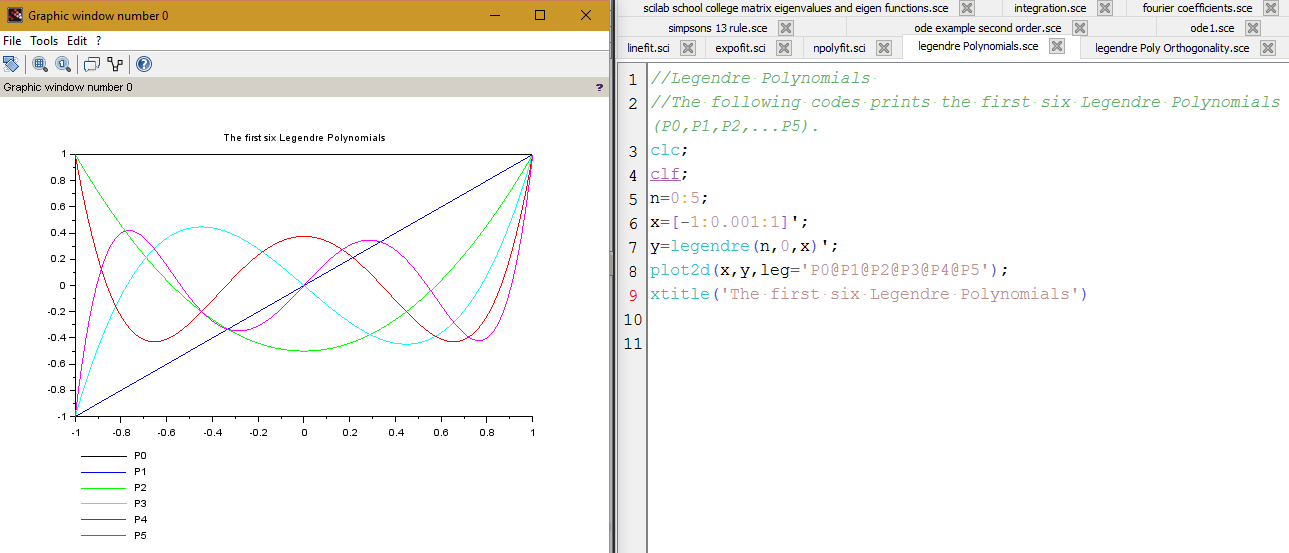# Plotting the First 6 LEGENDRE Polynomials using Scilab

To work with Legendre Polynomials we use the Scilab function legendre(n,m,x).

Which basically returns the value of the Associated Legendre Polynomial for a given value of m,n and x.
However, since I only wanted Legendre Polynomials so I’ll have to put m=0.

The following code calculates and plots the first six Legendre Polynomials for x=-1 to x=1.

```//Legendre Polynomials
//The following codes prints the first six Legendre Polynomials(P0,P1,P2,...P5).
clc;
clf;
n=0:5;
x=[-1:0.001:1]';
y=legendre(n,0,x)';
plot2d(x,y,leg='[email protected]@[email protected]@[email protected]');
xtitle('The first six Legendre Polynomials')
ylabel('Pn(x)');
xlabel('x');
```

The output:[wpedon id="7041" align="center"]

## 5 thoughts on “Plotting the First 6 LEGENDRE Polynomials using Scilab”

1. Thanks a lot for such insightful videos and explanations. These programs proved to be extremely useful. I can’t thank you enough.

1. Happy to help! Appreciate your feedback.

2. Can you help me ? I tried to obtain the plot of five first Hermite polynomial using Scilab but I failed. If someone know it, please send the code

1. I will try it if I get time. I can’t promise anything though, as I am very occupied these days.

3. Got some help with Legendre Polynomial. Thank you very much. Need some help with — Second order Differential Equation
• Harmonic oscillator (no friction)
• Damped Harmonic oscillator
• Over damped
• Critical damped
• Oscillatory
• Forced Harmonic oscillator
in scilab
thanks in advance. My mail is [email protected]